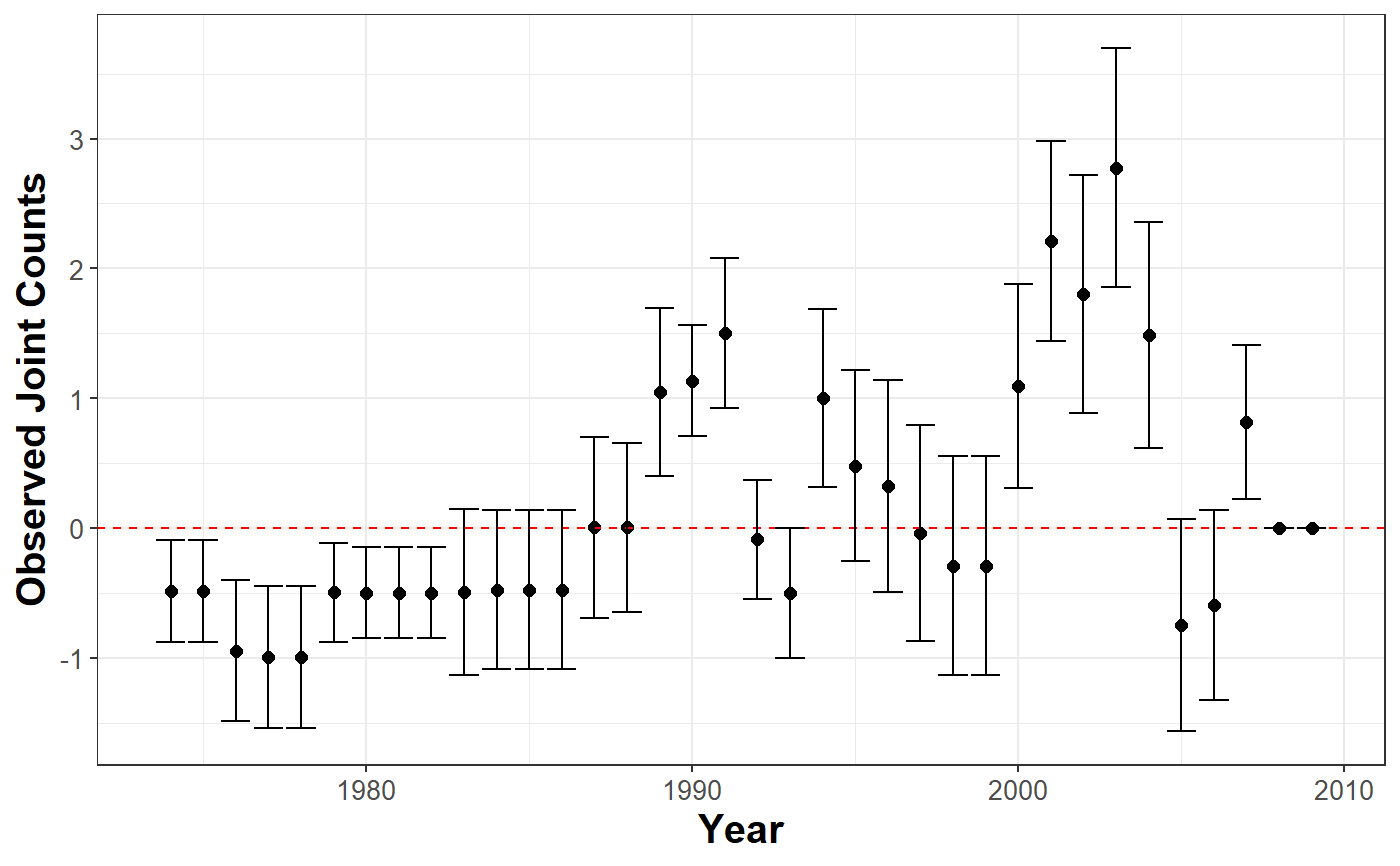Uses Joint Count tests to assess spatial clustering or dispersion of categorical variables in the data. Negative values indicate positive spatial clustering.

plot_JointCount(
data,
var_cured = character(),
var_id = character(),
var_time = character(),
n = 1,
t = 1.645,
threshold = 800L
)

## Arguments

data data. binary indicator of immunity. ID's unique identifier. variable that measures time. number of observation per id. value of the confidence interval. distance in kilometers. By default is 800.

A ggplot object

## Examples


library(BayesSPsurv)
dataw  <- spduration::add_duration(data = BayesSPsurv::Walter_2015_JCR,
y = "renewed_war",
unitID = "ccode",
tID = "year",
freq = "year",
ongoing = FALSE)#> Warning: Converting to 'Date' class with yyyy-06-30

plot_JointCount(data = dataw,
var_cured = "cured",
var_id = "ccode",
var_time = "year",
n = 12)